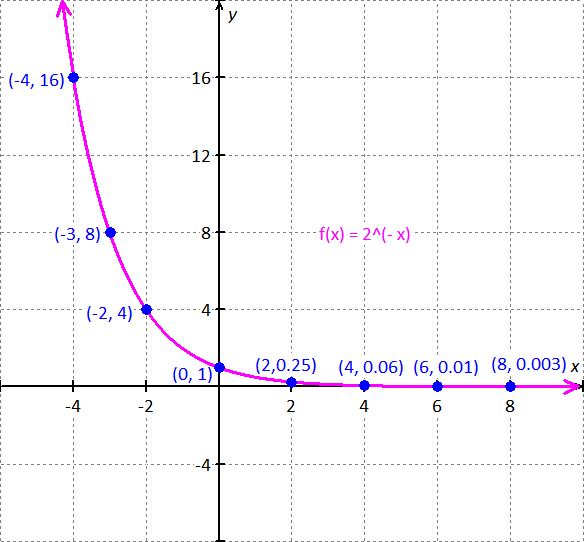PRE-ALGEBRA
ALGEBRA 1
GEOMETRY
ALGEBRA 2
TRIGONOMETRY
PRE-CALCULUS
CALCULUSPrecalculus 2014PAGE: 166 SET: Exercises PROBLEM: 1
Please look in your text book for this problem StatementThe function is .

Make the table of values to find ordered pairs that satisfy the function.

Choose values for  and find the corresponding values forGraph:

1. Draw a coordinate plane.

2. Plot the coordinate points.

3. Then sketch the graph, connecting the points with a smooth curve.Observe the above graph :

Domain of the function is all real numbers.

Range of the function is .

The function does not have the x-intercept.

- intercepts is .

The line  is the horizontal asymptote of the curve .

if either or .

.

is the horizontal asymptote of the function.

Observe the above graph the function does not have vertical asymptote.

End behavior :  and .

The function is continuous for all real numbers.

Decreasing on the interval : .The graph of the function  is :Domain of the function is all real numbers.

Range of the function is .

The function does not have any  -intercept.

- intercepts is

Horizontal asymptote of the function is .

End behavior :  and .

The function is decreasing over the interval : .TESTIMONIALS
 "I want to tell you that our students did well on the math exam and showed a marked improvement that, in my estimation, reflected the professional development the faculty received from you. THANK YOU!!!" June Barnett "Your site is amazing! It helped me get through Algebra." Charles "My daughter uses it to supplement her Algebra 1 school work. She finds it very helpful." Dan Pease
 Tweets by @mathskeydotcom

QUESTIONS? LET US HELP.
mathskey.com is not affiliated with any Publisher, Book cover, Title, Author names appear for reference only